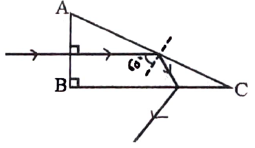Request a call back

# ICSE Class 10 Physics Previous Year Question Paper Semester 1 2021

In ICSE Class 10, Physics is one of the most important subjects. The fundamentals learnt also assist students in clearing the competitive exams like IIT-JEE and NEET. TopperLearning provides ICSE Class 10 Physics students with robust study materials to help them score well in the examination. Our study materials are created by subject experts and provide better insight into preparing for the ICSE Class 10 Physics examination.

Students utilize the online ICSE Class 10 Tests as a practice tool to enhance their exam preparation strategies. Experienced and qualified faculty members design the tests available in concept, chapter and multiple chapter-wise. Students can access these tests and other resources anytime to boost their practice and revision aids.

Students are recommended to go through the content resources available on the website and study the crucial resources available.

We recommend that users subscribe to TopperLearning packs from ICSE Class 10 Plans.

Question 1.

The deviation produced by an equilateral prism does not depend on:                                      

1. the angle of incidence.
2. the size of the prism.
3. the material of the prism.
4. the colour of light used.

Question 2.

The refractive index of a diamond is 2.4. It means that:                                                       

1. the speed of light in vacuum is equal to   times the speed of light in diamond.
2. the speed of light in the diamond is 2.4 times the speed of light in a vacuum.
3. the speed of light in a vacuum is 2.4 times the speed of light in the diamond.
4. the wavelength of light in diamond is 2.4 times the wavelength of light in vacuum.

Question 3.

An object of height 10 cm is placed in front of a concave lens of focal length 20 cm at a distance 25 cm from the lens. Is it possible to capture this image on a screen? Select a correct option from the following:                                                                    

1. Yes, as the image formed will be real.
2. Yes, as the image formed will be erect.
3. No, as the image formed will be virtual.
4. No, as the image formed will be inverted.

Question 4.

A ray of light IM is incident on a glass slab ABCD as shown in the figure below. The emergent ray for this incident ray is:                

1. NQ
2. NR
3. NP
4. NS

Question 5.

The colour of white light which is deviated least by a prism is:                                                                   

1. green
2. yellow
3. red
4. violet

Question 6.

The wave length range of visible light is:                                                                                                 

1. 40 nm to 80 nm
2. 4000 nm to 8000 nm
3. 4 nm to 8 nm
4. 400 nm to 800 nm

Question 7.

Observe the diagram which shows the path of an incident ray through an optical plane LL" of a lens. The focal length of the lens is 20 cm.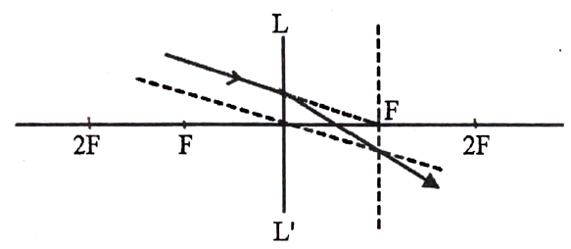(i) If an object is placed at a distance of 30 cm in front of this lens, then:                                

1. the image will be virtual
2. the image will be diminished and inverted.
3. the image will be diminished.
4. the image will be real and magnified.

(ii) This type of lens can be used:                                                                                        

1. to correct hypermetropia.
2. to correct myopia.
3. to diverge light.
4. in the front door peepholes.

(iii) An object is placed in front of this lens at a distance of 60 cm. Then the image distance from the lens with proper sign convention is:       

1. +60 cm
2. +30 cm
3. -30 cm
4. +15 cm

(iv) An object is placed in front of this lens at a distance of 60 cm. Then the magnification of the image is:                                           

1. 0.25
2. 1.25
3. -0.5
4. 1

Question 8.

The relation between CGS and S.I. unit of moment of force is:                                   

1. 1 Nm= 105 dyne cm
2. 1 Nm = 105 dyne
3. 1 Nm= 107 dyne cm
4. 1 dyne cm = 107 N m

Question 9.

A coolie raises a load upwards against the force of gravity then the work done by the load is:     

1. zero.
2. positive work.
3. negative work.
4. none of these.

Question 10.

The energy change during photosynthesis in plants is:                      

1. heat to chemical.
2. light to chemical.
3. chemical to light.
4. chemical to heat.

Question 11.

The diagram below shows the balanced position of a metre scale.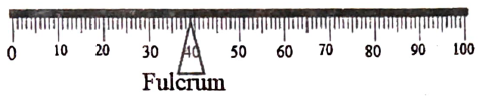Which one of the following diagrams shows the correct position of the scale when it is supported at the centre?

(a)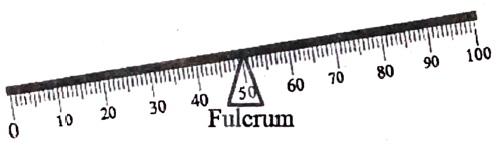(b)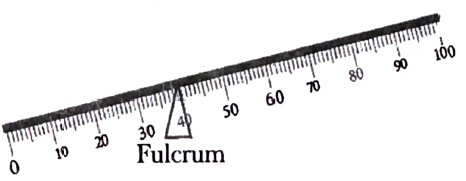(c)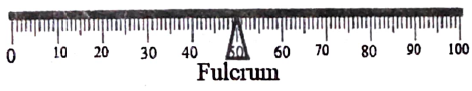(d)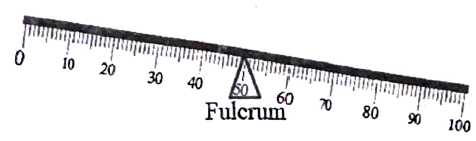Question 12.

A stone tied at the end of a string is whirled by hand in a horizontal circle with uniform speed.

(i) Name the force required for this circular motion:                   

1. Centrifugal force.
2. Centripetal force.
3. Force of gravity.
4. Frictional force.

(ii) What is the direction of the above-mentioned force?              

1. Towards the centre of the circular path.
2. Away from the centre of the circular path.
3. Normal to the radius at a point where the body is present on the circular path.
4. Direction of this force keeps on changing alternately towards and away from the centre.

Question 13.

A body of mass 200 g falls freely from a height of 15 m. [g = 10 ms-2]

(i) When the body reaches 10 m above the ground, its potential energy will be:                           

1. 20000 J
2. 10 J
3. 10000 J
4. 20 J

(ii) The gain in kinetic energy of the body when it reaches 10 m above the ground is:                    

1. 20 J
2. 10 J
3. 30 J
4. 25 J

(iii) The total mechanical energy it will possess, when it is just about to strike the ground is:           

1. 30000 J
2. 20000 J
3. 30 J
4. 20 J

(iv) The velocity in ms-1 with which the body will hit the ground is:                                          

1. 30
2. 10
3. 10√3
4. 10√2

Question 14.

A woman draws water from a well using a fixed pulley. The mass of the bucket and the water together is 10 kg. The force applied by the woman is 200 N. The mechanical advantage is (g = 10 m/s2):                                                              

1. 2
2. 20
3. 0.05
4. 0.5

Question 15.

A single fixed pulley is used because:                                                   

1. it changes the direction of applied effort conveniently.
2. it multiplies speed.
3. it multiplies effort.
4. its efficiency is 100%.

Question 16.

In the diagram shown below, the velocity ratio of the arrangement is: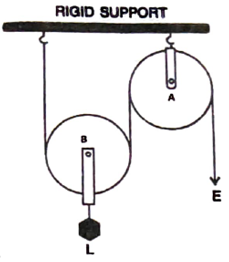1. 1
2. 2
3. 3
4. 0

Question 17.

Which one of the following is the correct mathematical relation?                   

1. Power = Force/ Velocity
2. Power = Force x Acceleration
3. Power Force/ Acceleration
4. Power= Force x Velocity

Question 18.

Select a correct option with respect to echo depth sounding:                       

1. infrasonic waves are used
2. the frequency of the waves used is between 20 Hz and 20,000 Hz.
3. ultrasonic waves are used.
4. supersonic waves are used.

Question 19.

Which one of the following diagnostic methods use reflection of sound?          

1. CT scan
2. Electrocardiogram
3. Echo cardiogram
4. MRI

Question 20.

A boy standing in front of a wall produces two whistles per second. He notices that the sound of his whistling coincides with the echo. The echo is heard only once when whistling is stopped. Calculate the distance between the boy and the wall. (The speed of sound in air = 320 m/s).

(i) The time in which the boy hears the echo is:            

1. 1 s
2. 0.5 s
3. 1.5 s
4. 2 s

(ii)The distance at which the boy is standing from the wall:                     

1. 160 m
2. 240 m
3. 320 m
4. 80 m

(iii) If the speed of sound is increased by 16 ms-1 and the boy moves 4 m away from the wall then in how much time will he hear the echo of the first whistle?   

1. 0.525 s
2. 0.5 s
3. 0.48 s
4. 0.3 s

(iv) In which of the following timings of reflection of the whistle, the echo cannot be heard?    

1. 0.05 s
2. 0.12 s
3. 0.2 s
4. 0.11 s

Question 21.

The ratio of velocities of light of wavelength 400 nm and 800 nm in a vacuum is: 

1. 1:1
2. 1:2
3. 2:1
4. 1:3

Question 22.

1 joule= __________ erg           

1. 109
2. 107
3. 105
4. 106

Question 23.

A light body A and a heavy body B have the same momentum.

(i) Choose a correct statement from the given options.            

1. kinetic energy of body A and body B will be the same.
2. kinetic energy of body A is greater than kinetic energy of body B.
3. kinetic energy of body B is greater than kinetic energy of body A.
4. unless we know the velocity, we cannot find which body has greater kinetic energy.

(ii)If the ratio of kinetic energies of A and B is 5:2 then which one of the following gives the mass ratio of the bodies respectively?     

1. 5 : 2
2. 25 : 4
3. 2 : 5
4. 4 : 24

Question 24.

The diagram below shows a ray of light travelling from air into a glass material as shown below. Answer the questions that follow: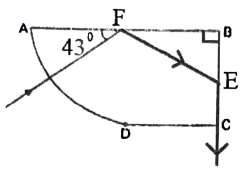(i) The angle of incidence at the surface AB is:      

1. 43o
2. 47o
3. 90o
4. 0o

(ii) Select a correct statement from the following.                           

1. The speed of light at the curved surface AD does not change while entering the block.
2. The ray at the surface AD is not travelling along the radius of the curved part
3. The ray at the surface AD is travelling along the radius of the curved part.
4. Light never refracts when it enters a curved surface.

(iii) The angle of incidence on the surface BC is:                

1. 43o
2. 47o
3. 90o
4. 0o

(iv) The critical angle of this material of glass:                    

1. 47o
2. 43o
3. 42o
4. 45o

Question 25.

The diagram below shows the path of light passing through a right-angled prism of critical angle 42o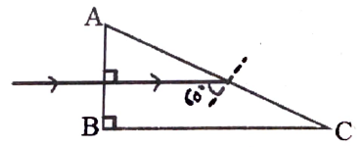(i) The angle C of the prism is:     

1. 45o
2. 60o
3. 90o
4. 30o

(ii) Which one of the following diagrams shows the correct path of this ray till it emerges out of the prism?          

 (a)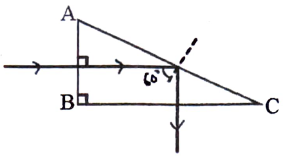(b)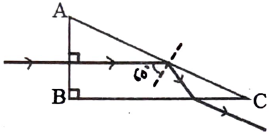(c)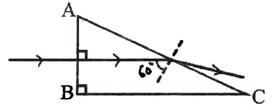(d)